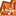# App Development

Announcements
Support Cases coming to Qlik Community Oct. 4! Start chats, open cases, explore resources: READ DETAILS
cancel
Showing results for
Did you mean:Contributor

## Variable used in set analysis won't slice by dimension

Hello,

I've been all over the forums and looking through training documentation including the 600+ page reference manual for Qlik Sense.  I understand that when I create a variable in the front end I have the option of using = at the beginning, and it will control how the expression evaluates.

eg: a variable with the definition Sum(Sales) will slice by dimensions in a chart, while =Sum(Sales) will simply show the total sales regardless of the dimensions in a chart.

I have the variable vSPPM, which without the equals sign in front of it will slice by dimensions in tables and charts:

``````(Sum({<Forecast,[%Prior6MonthFlag]={'1'}>}Rejects)/
(Sum({<Forecast,[%Prior6MonthFlag]={'1'}>}Receipts)-0.0000001))*1000000``````Of course putting the equals sign in front of the formula above would produce the undesired result where all regions would show the total value of 15.7186. But that's not the issue since we can see the table is properly slicing the variable by regions above.

I then call the variable inside a set expression inside another variable vGetPtsSPPM:

``````SUM({\$ <
[Metric Group] = {'Scoring'}
,[Child Metric Name] = {'PPM'}
,[Max] = {">\$(=\$(vSPPM))"}
,[Min] = {"<=\$(=\$(vSPPM))"}
>}
Points)``````

The expression above goes to a table to retrieve points based on a Min and Max column.  I need to return the points where the values in the table above are between min and max for a particular metric.  I've tried every possible combination of dollar, equals, parenthesis I can think of, and the only one that semi-works is above.  The problem is that vSPPM always evaluates to the total of 15.71... instead of slicing in the table by region.  So the result I'm looking to calculate is to have the variable evaluate in the table by region.  For example, the row where Region = R3 it should pass the 10.041 seen above.  Instead it always passes in the total of 15.7186...

Labels (3)

• ### Variables

1 Solution

Accepted SolutionsLuminary Alumni

What you are trying to do isn't possible. Qlik's evaluation steps are

1. Dollar Sign Expansion (from inner most to outermost),
2. Set Analysis: Defines the data available to expression in the hypercube
3. Expression on top of the resulting Set Analysis defined dataset over the Dimensions.

So you can't leverage dimensionality within Set Analysis. The only way you can do it is using the AGGR function within the inner set analysis function and defining the dimensions in the expression itself.Luminary Alumni

What you are trying to do isn't possible. Qlik's evaluation steps are

1. Dollar Sign Expansion (from inner most to outermost),
2. Set Analysis: Defines the data available to expression in the hypercube
3. Expression on top of the resulting Set Analysis defined dataset over the Dimensions.

So you can't leverage dimensionality within Set Analysis. The only way you can do it is using the AGGR function within the inner set analysis function and defining the dimensions in the expression itself.